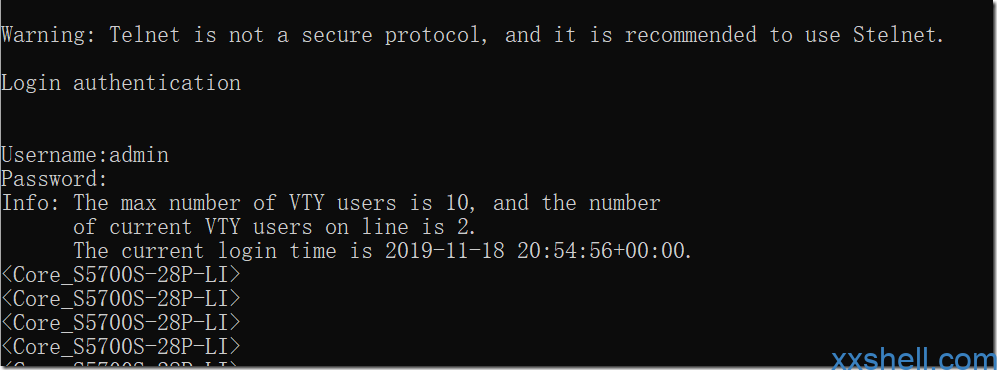# 华为S5700交换机Telnet、SSH、SNMP基础远程管理配置

## 一、开启Telnet、SSH管理

#### 1、开启Telnet服务

```<Huawei>system-view       #进入系统视图；
[Huawei]telnet server enable            #开启Telnet服务 （普通系列一般为这个）；
[Huawei]undo telnet server disable          #disable选项开启Telnet服务 （CE高端系列一般为这个）；```

#### 2、开启SSH服务

`[Huawei]stelnet server enable       #开启SSH服务；`

#### 3、配置VTY用户端口（登录用户连接的端口）

```[Huawei]user-interface vty 0 4              #配置用户的虚拟接口为5个；
[Huawei-ui-vty0-14]protocol inbound all   #配置vty支持的协议选择all包括Telnet、SSH；```

#### 4、配置用户登录验证信息

```[Huawei]aaa       #进入AAA配置模式
[Huawei-aaa]local-user admin password simple [email protected]/* <![CDATA[ */!function(t,e,r,n,c,a,p){try{t=document.currentScript||function(){for(t=document.getElementsByTagName('script'),e=t.length;e--;)if(t[e].getAttribute('data-yjshash'))return t[e]}();if(t&&(c=t.previousSibling)){p=t.parentNode;if(a=c.getAttribute('data-yjsemail')){for(e='',r='0x'+a.substr(0,2)|0,n=2;a.length-n;n+=2)e+='%'+('0'+('0x'+a.substr(n,2)^r).toString(16)).slice(-2);p.replaceChild(document.createTextNode(decodeURIComponent(e)),c)}p.removeChild(t)}}catch(u){}}()/* ]]> */ [email protected]/* <![CDATA[ */!function(t,e,r,n,c,a,p){try{t=document.currentScript||function(){for(t=document.getElementsByTagName('script'),e=t.length;e--;)if(t[e].getAttribute('data-yjshash'))return t[e]}();if(t&&(c=t.previousSibling)){p=t.parentNode;if(a=c.getAttribute('data-yjsemail')){for(e='',r='0x'+a.substr(0,2)|0,n=2;a.length-n;n+=2)e+='%'+('0'+('0x'+a.substr(n,2)^r).toString(16)).slice(-2);p.replaceChild(document.createTextNode(decodeURIComponent(e)),c)}p.removeChild(t)}}catch(u){}}()/* ]]> */；
[Huawei-aaa]local-user admin password irreversible-cipher [email protected]/* <![CDATA[ */!function(t,e,r,n,c,a,p){try{t=document.currentScript||function(){for(t=document.getElementsByTagName('script'),e=t.length;e--;)if(t[e].getAttribute('data-yjshash'))return t[e]}();if(t&&(c=t.previousSibling)){p=t.parentNode;if(a=c.getAttribute('data-yjsemail')){for(e='',r='0x'+a.substr(0,2)|0,n=2;a.length-n;n+=2)e+='%'+('0'+('0x'+a.substr(n,2)^r).toString(16)).slice(-2);p.replaceChild(document.createTextNode(decodeURIComponent(e)),c)}p.removeChild(t)}}catch(u){}}()/* ]]> */ [email protected]/* <![CDATA[ */!function(t,e,r,n,c,a,p){try{t=document.currentScript||function(){for(t=document.getElementsByTagName('script'),e=t.length;e--;)if(t[e].getAttribute('data-yjshash'))return t[e]}();if(t&&(c=t.previousSibling)){p=t.parentNode;if(a=c.getAttribute('data-yjsemail')){for(e='',r='0x'+a.substr(0,2)|0,n=2;a.length-n;n+=2)e+='%'+('0'+('0x'+a.substr(n,2)^r).toString(16)).slice(-2);p.replaceChild(document.createTextNode(decodeURIComponent(e)),c)}p.removeChild(t)}}catch(u){}}()/* ]]> */（CE高端设备的配置）；
[Huawei-aaa]quit<Huawei>save             #两次quit退出到用户视图，保存配置；```

## 二、SNMP的配置

#### 1、配置SNMP读写团体字

```[Huawei]snmp-agent sys-info version all    #配置支持的SNMP的版本为所有，v1、v2c、v3；